Home | | Maths 12th Std | Indeterminate Forms

# Indeterminate Forms

In this section, we shall discuss various ŌĆ£indeterminate formsŌĆØ and methods of evaluating the limits when we come across them.

Indeterminate Forms

In this section, we shall discuss various ŌĆ£indeterminate formsŌĆØ and methods of evaluating the limits when we come across them.

## A Limit Process

While computing the limitslim xŌåÆ╬▒ R(x)

of certain functions R( x) , we may come across the following situations like,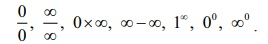We say that they have the form of a number. But values cannot be assigned to them in a way that is consistent with the usual rules of addition and mutiplication of numbers. We call these expressions indeterminate forms. Although they are not numbers, these indeterminate forms play a useful role in the limiting behaviour of a function.

John (Johann) Bernoulli discovered a rule using derivatives to compute the limits of fractions whose numerators and denominators both approach zero or Ōł×. The rule is known today as lŌĆÖH├┤pitalŌĆÖs Rule (pronounced as Lho pi tal Rule), named after Guillaume de lŌĆÖHospitalŌĆÖs, a French nobleman who wrote the earliest introductory differential calculus text, where the rule first appeared in print.

## The lŌĆÖH├┤pitalŌĆÖs Rule

Suppose f ( x) and g ( x) are differentiable functions and g(xŌĆ▓) ŌēĀ 0 with## Indeterminate forms  0/0, Ōł×/Ōł×, 0├ŚŌł×, Ōł×-Ōł×

Example 7.33Solution

If we put directly x = 1 we observe that the given function is in an indeterminate form 0/0. As the numerator and the denominator functions are polynomials of degree 2 they both are differentiable. Hence, by an application of the lŌĆÖH├┤pital Rule, we getNote that this limit may also be evaluated through the factorization of the numerator and denominator asExample 7.34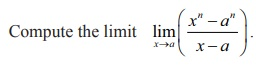Solution

If we put directly x =a we observe that the given function is in an indeterminate form 0/0 . As the numerator and the denominator functions are polynomials they both are differentiable.

Hence by an application of the lŌĆÖH├┤pital Rule we get,Example 7.35Solution

If we directly substitute x = 0 we get an indeterminate form  0/0 and hence we apply the lŌĆÖH├┤pitalŌĆÖs rule to evaluate the limit as,The next example tells that the limit does not exist.

Example 7.36Solution

If we directly substitute x = 0 we get an indeterminate form 0/0 and hence we apply the lŌĆÖH├┤pitalŌĆÖs rule to evaluate the limit as,As the left limit and the right limit are not the same we conclude that the limit does not exist.

Remark

One may be tempted to use the lŌĆÖH├┤pitalŌĆÖs rule once again inwhich is not true because it was not an indeterminate form.

Example 7.37Solution

As this is an indeterminate form (0/0), using the lŌĆÖH├┤pitalŌĆÖs Rule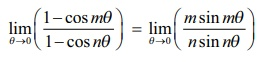Now using the example 7.35, we have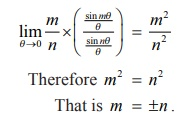Example 7.38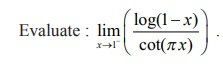Solution

This is an indeterminate form Ōł×/Ōł× and hence we use the lŌĆÖH├┤pitalŌĆÖs Rule to evaluate the limit.Example 7.39Solution

This is an indeterminate of the form Ōł× ŌłÆ Ōł× . To evaluate this limit we first simplify and bring it in the form (0/0) and applying the lŌĆÖH├┤pital Rule, we getExample 7.40

Evaluate :Solution

This is an indeterminate of the form (0 ├Ś Ōł×) . To evaluate this limit, we first simplify and bring it to the form (Ōł×/Ōł×) and apply lŌĆÖH├┤pital Rule. Thus, we getExample 7.41Solution

This is an indeterminate of the form (Ōł×/Ōł×) . To evaluate this limit, we apply lŌĆÖH├┤pital Rule.Example 7.42Solution

This is an indeterminate of the form (Ōł×/Ōł×).

To evaluate this limit, we apply lŌĆÖH├┤pital Rule m times.

Thus, we have

limxŌåÆŌł× ex/xm  = limxŌåÆŌł× ex/m!

= Ōł× .## Indeterminate forms 00,1Ōł× and Ōł×0

In order to evaluate the indeterminate forms like this, we shall first state the theorem on the limit of a composite function.

Theorem 7.6

Let limxŌåÆ╬▒ g ( x) exist and let it be L and let f (x) be a continuous function at x = L . Then,## The evaluation procedure for evaluating the limits

(1) Let A = limxŌåÆ╬▒ g(x) . Then taking logarithm, with the assumption that A > 0 to ensure the continuity of the logarithm function, we get log A = limxŌåÆ╬▒ log(g(x)) . Therefore using the above theorem with f (x) = log x we have the limit(2) We have the limit lim xŌåÆ╬▒ log(g ( x)) into either (0/0) or (Ōł×/Ōł×) form evaluate it using lŌĆÖH├┤pital Rule.

(3) Let that evaluated limit be say ╬▒ . Then the required limit is e╬▒ .

Example 7.43

Using the lŌĆÖH├┤pital Rule, prove that limxŌåÆ╬▒ (1+ x )1/x = e .

Solution

This is an indeterminate of the form 1Ōł× . Let g ( x) = (1+ x)1/x. Taking the logarithm, we get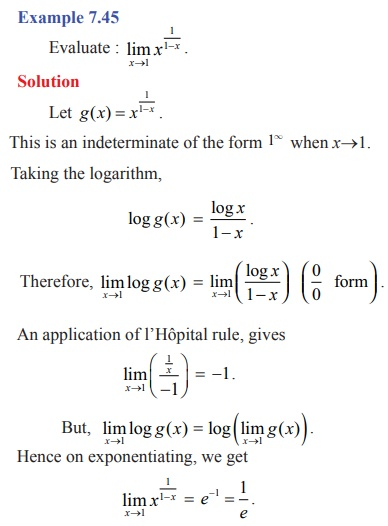Tags : Applications of Differential Calculus | Mathematics , 12th Maths : UNIT 7 : Applications of Differential Calculus
Study Material, Lecturing Notes, Assignment, Reference, Wiki description explanation, brief detail
12th Maths : UNIT 7 : Applications of Differential Calculus : Indeterminate Forms | Applications of Differential Calculus | Mathematics